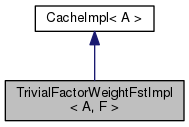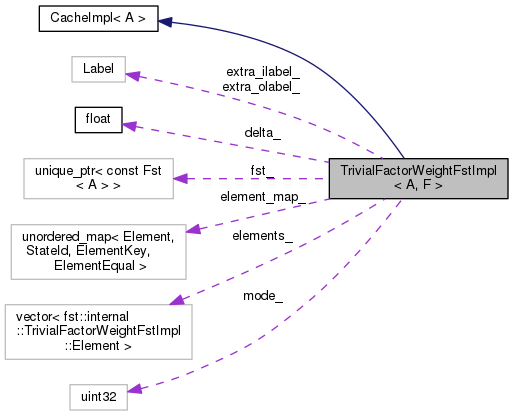TrivialFactorWeightFstImpl< A, F > Class Template Reference

`#include <trivial-factor-weight.h>`

Inheritance diagram for TrivialFactorWeightFstImpl< A, F >:[legend]
Collaboration diagram for TrivialFactorWeightFstImpl< A, F >:[legend]

## Classes

struct  Element

class  ElementEqual

class  ElementKey

## Public Types

typedef A Arc

typedef A::Label Label

typedef A::Weight Weight

typedef A::StateId StateId

typedef F FactorIterator

typedef DefaultCacheStore< A > Store

typedef Store::State State

## Public Member Functions

TrivialFactorWeightFstImpl (const Fst< A > &fst, const TrivialFactorWeightOptions< A > &opts)

TrivialFactorWeightFstImpl (const TrivialFactorWeightFstImpl< A, F > &impl)

StateId Start ()

Weight Final (StateId s)

size_t NumArcs (StateId s)

size_t NumInputEpsilons (StateId s)

size_t NumOutputEpsilons (StateId s)

void InitArcIterator (StateId s, ArcIteratorData< A > *data)

StateId FindState (const Element &e)

void Expand (StateId s)

## Private Types

typedef unordered_map< Element, StateId, ElementKey, ElementEqualElementMap

## Private Attributes

std::unique_ptr< const Fst< A > > fst_

float delta_

uint32 mode_

Label extra_ilabel_

Label extra_olabel_

std::vector< Elementelements_

ElementMap element_map_

## Detailed Description

### template<class A, class F> class fst::internal::TrivialFactorWeightFstImpl< A, F >

Definition at line 92 of file trivial-factor-weight.h.

## ◆ Arc

 typedef A Arc

Definition at line 106 of file trivial-factor-weight.h.

## ◆ ElementMap

 typedef unordered_map ElementMap
private

Definition at line 294 of file trivial-factor-weight.h.

## ◆ FactorIterator

 typedef F FactorIterator

Definition at line 110 of file trivial-factor-weight.h.

## ◆ Label

 typedef A::Label Label

Definition at line 107 of file trivial-factor-weight.h.

## ◆ State

 typedef Store::State State

Definition at line 113 of file trivial-factor-weight.h.

## ◆ StateId

 typedef A::StateId StateId

Definition at line 109 of file trivial-factor-weight.h.

## ◆ Store

 typedef DefaultCacheStore Store

Definition at line 112 of file trivial-factor-weight.h.

## ◆ Weight

 typedef A::Weight Weight

Definition at line 108 of file trivial-factor-weight.h.

## ◆ TrivialFactorWeightFstImpl() [1/2]

 TrivialFactorWeightFstImpl ( const Fst< A > & fst, const TrivialFactorWeightOptions< A > & opts )
inline

Definition at line 124 of file trivial-factor-weight.h.

125  : CacheImpl<A>(opts),
126  fst_(fst.Copy()),
127  delta_(opts.delta),
128  extra_ilabel_(opts.extra_ilabel),
129  extra_olabel_(opts.extra_olabel) {
130  SetType("factor-weight");
131  uint64 props = fst.Properties(kFstProperties, false);
132  SetProperties(FactorWeightProperties(props), kCopyProperties);
133
134  SetInputSymbols(fst.InputSymbols());
135  SetOutputSymbols(fst.OutputSymbols());
136  }
For an extended explanation of the framework of which grammar-fsts are a part, please see Support for...
Definition: graph.dox:21
std::unique_ptr< const Fst< A > > fst_

## ◆ TrivialFactorWeightFstImpl() [2/2]

 TrivialFactorWeightFstImpl ( const TrivialFactorWeightFstImpl< A, F > & impl )
inline

Definition at line 138 of file trivial-factor-weight.h.

139  : CacheImpl<A>(impl),
140  fst_(impl.fst_->Copy(true)),
141  delta_(impl.delta_),
142  extra_ilabel_(impl.extra_ilabel_),
143  extra_olabel_(impl.extra_olabel_) {
144  SetType("factor-weight");
145  SetProperties(impl.Properties(), kCopyProperties);
146  SetInputSymbols(impl.InputSymbols());
147  SetOutputSymbols(impl.OutputSymbols());
148  }
std::unique_ptr< const Fst< A > > fst_

## ◆ Expand()

 void Expand ( StateId s )
inline

Definition at line 230 of file trivial-factor-weight.h.

230  {
231  CHECK(static_cast<size_t>(s) < elements_.size());
232  Element e = elements_[s];
233  if (e.weight != Weight::One()) {
234  FactorIterator fit(e.weight);
235  if (fit.Done()) { // Cannot be factored-> create a link to dest state directly
236  if (e.state != kNoStateId) {
237  StateId dest = FindState(Element(e.state, Weight::One()));
238  PushArc(s, Arc(extra_ilabel_, extra_olabel_, e.weight, dest));
239  } // else we're done. This is a final state.
240  } else { // Can be factored.
241  const std::pair<Weight, Weight> &p = fit.Value();
242  StateId dest = FindState(Element(e.state, p.second.Quantize(delta_)));
243  PushArc(s, Arc(extra_ilabel_, extra_olabel_, p.first, dest));
244  }
245  } else { // Unit weight. This corresponds to a "real" state.
246  CHECK(e.state != kNoStateId);
247  for (ArcIterator< Fst<A> > ait(*fst_, e.state);
248  !ait.Done();
249  ait.Next()) {
250  const A &arc = ait.Value();
251  FactorIterator fit(arc.weight);
252  if (fit.Done()) { // cannot be factored->just link directly to dest.
253  StateId dest = FindState(Element(arc.nextstate, Weight::One()));
254  PushArc(s, Arc(arc.ilabel, arc.olabel, arc.weight, dest));
255  } else {
256  const std::pair<Weight, Weight> &p = fit.Value();
257  StateId dest = FindState(Element(arc.nextstate, p.second.Quantize(delta_)));
258  PushArc(s, Arc(arc.ilabel, arc.olabel, p.first, dest));
259  }
260  }
261  // See if we have to add arcs for final-states [only if final-weight is factorable].
262  Weight final_w = fst_->Final(e.state);
263  if (final_w != Weight::Zero()) {
264  FactorIterator fit(final_w);
265  if (!fit.Done()) {
266  const std::pair<Weight, Weight> &p = fit.Value();
267  StateId dest = FindState(Element(kNoStateId, p.second.Quantize(delta_)));
268  PushArc(s, Arc(extra_ilabel_, extra_olabel_, p.first, dest));
269  }
270  }
271  }
272  this->SetArcs(s);
273  }
std::unique_ptr< const Fst< A > > fst_

## ◆ Final()

 Weight Final ( StateId s )
inline

Definition at line 161 of file trivial-factor-weight.h.

161  {
162  if (!HasFinal(s)) {
163  const Element &e = elements_[s];
164  Weight w;
165  if (e.state == kNoStateId) { // extra state inserted to represent final weights.
166  FactorIterator fit(e.weight);
167  if (fit.Done()) { // cannot be factored.
168  w = e.weight; // so it's final
169  } else {
170  w = Weight::Zero(); // need another transition.
171  }
172  } else {
173  if (e.weight != Weight::One()) { // Not a real state.
174  w = Weight::Zero();
175  } else { // corresponds to a "real" state.
176  w = fst_->Final(e.state);
177  FactorIterator fit(w);
178  if (!fit.Done()) // we would have intermediate states representing this final state.
179  w = Weight::Zero();
180  }
181  }
182  this->SetFinal(s, w);
183  return w;
184  } else {
185  return CacheImpl<A>::Final(s);
186  }
187  }
std::unique_ptr< const Fst< A > > fst_

## ◆ FindState()

 StateId FindState ( const Element & e )
inline

Definition at line 216 of file trivial-factor-weight.h.

216  {
217  typename ElementMap::iterator eit = element_map_.find(e);
218  if (eit != element_map_.end()) {
219  return (*eit).second;
220  } else {
221  StateId s = elements_.size();
222  elements_.push_back(e);
223  element_map_.insert(std::pair<const Element, StateId>(e, s));
224  return s;
225  }
226  }

## ◆ InitArcIterator()

 void InitArcIterator ( StateId s, ArcIteratorData< A > * data )
inline

Definition at line 207 of file trivial-factor-weight.h.

207  {
208  if (!HasArcs(s))
209  Expand(s);
211  }

## ◆ NumArcs()

 size_t NumArcs ( StateId s )
inline

Definition at line 189 of file trivial-factor-weight.h.

References fst::NumArcs().

189  {
190  if (!HasArcs(s))
191  Expand(s);
192  return CacheImpl<A>::NumArcs(s);
193  }
Arc::StateId NumArcs(const ExpandedFst< Arc > &fst)
Returns the total number of arcs in an FST.

## ◆ NumInputEpsilons()

 size_t NumInputEpsilons ( StateId s )
inline

Definition at line 195 of file trivial-factor-weight.h.

195  {
196  if (!HasArcs(s))
197  Expand(s);
199  }

## ◆ NumOutputEpsilons()

 size_t NumOutputEpsilons ( StateId s )
inline

Definition at line 201 of file trivial-factor-weight.h.

201  {
202  if (!HasArcs(s))
203  Expand(s);
205  }

## ◆ Start()

 StateId Start ( )
inline

Definition at line 150 of file trivial-factor-weight.h.

150  {
151  if (!HasStart()) {
152  StateId s = fst_->Start();
153  if (s == kNoStateId)
154  return kNoStateId;
155  StateId start = this->FindState(Element(fst_->Start(), Weight::One()));
156  this->SetStart(start);
157  }
158  return CacheImpl<A>::Start();
159  }
std::unique_ptr< const Fst< A > > fst_

## ◆ delta_

 float delta_
private

Definition at line 297 of file trivial-factor-weight.h.

## ◆ element_map_

 ElementMap element_map_
private

Definition at line 302 of file trivial-factor-weight.h.

## ◆ elements_

 std::vector elements_
private

Definition at line 301 of file trivial-factor-weight.h.

## ◆ extra_ilabel_

 Label extra_ilabel_
private

Definition at line 299 of file trivial-factor-weight.h.

## ◆ extra_olabel_

 Label extra_olabel_
private

Definition at line 300 of file trivial-factor-weight.h.

## ◆ fst_

 std::unique_ptr > fst_
private

Definition at line 296 of file trivial-factor-weight.h.

## ◆ mode_

 uint32 mode_
private

Definition at line 298 of file trivial-factor-weight.h.

The documentation for this class was generated from the following file: